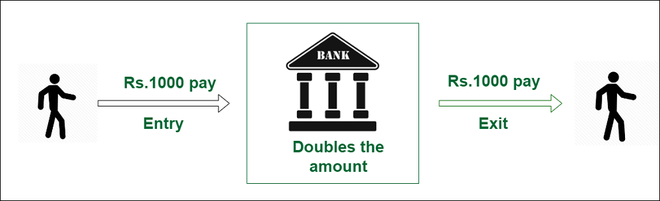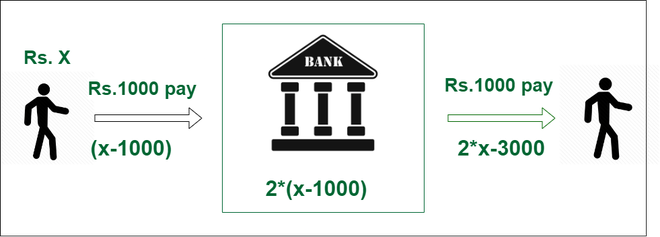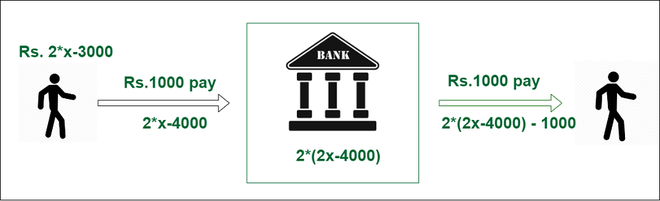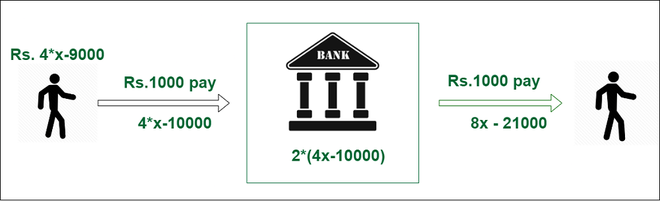# Puzzle | How much money did the man have before entering the bank?

Problem Statement:

Suppose a man had some amount in his wallet, he knows a bank that doubles the money immediately but the fee at the entrance of the bank is Rs 1000 and Rs 1000 as the exit fee. The man visited 3 branches of the same bank and when he left the last bank he had Rs 0 in his wallet. Find out what was the initial amount?Solution:

Let’s start from the end:

Step1: If he does not go to the third bank then the money left with him:

He gave 1000 rupees as exit fee on the third bank, so take 1000 rupees back so amount becomes 1000 and inside the bank, it will become half so 500 rupees now and take the entering fee also so, the amount becomes 1500 before going to the third branch of the bank.

Step2: If he does not go to the second bank then the money left with him:

He gave 1000 rupees as exit fee on the second bank so, take 1000 rupees back so amount becomes 2500 and inside the bank, it will become half so 1250 rupees now and also take the entry fee of 1000 rupees. so, the amount becomes 2250 before going to the second branch bank.

Step3:  If he does not go to the first bank then the money left with him:

He gave 1000 rupees as exit fee on the second bank so, take 1000 rupees back so amount becomes 3250 and inside the bank, it will become half so 1625 rupees now take the entry fee of 1000 rupees. so, the amount becomes 2625 before going to the first branch bank.

Hence he had 2625 rupees before visiting the bank.

Mathematical Approach:

Let’s take the initial amount he has as x:

At the first bank:• Subtract 1000 rupees as the entry fee so the amount becomes (x – 1000)
• Now, this amount becomes double inside the back. so, the new amount becomes 2*(x – 1000)
• Subtract 1000 rupees as the exit fee. so, the amount becomes 2*(x – 1000) – 1000
• Finally, the amount after visiting the first bank is (2*x – 3000)

At the second bank:• Subtract 1000 rupees as entry fee so amount becomes (2*x – 3000) – 1000 = (2*x – 4000)
• Now, this amount becomes double inside the back. so, the new amount becomes 2*( 2*x – 4000)
• Subtract 1000 rupees as the exit fee. so, the amount becomes 2*(2*x – 4000) – 1000
• Amount after visiting the second bank is (4*x – 9000)

At the third bank:• Subtract 1000 rupees as entry fee so amount becomes (4*x – 9000) – 1000 = (4*x – 10000)
• Now, this amount becomes double inside the back. so, the new amount becomes 2×(4*x – 10000)
• Subtract 1000 rupees as the exit fee. so, the amount becomes 2×(4*x – 10000) – 1000
• Amount after visiting a third bank is (8*x – 21000)

It is given that he had 0 rupees left after coming out from the third bank:

so,  8*x – 21000 = 0
8*x = 21000
x = 21000 / 8
x = 2625

Therefore, Initially, he had 2625 rupees in his wallet.